# KSEEB Solutions for Class 8 Maths Chapter 9 Commercial Arithmetic Ex 9.6

In this chapter, we provide KSEEB SSLC Class 8 Maths Chapter 9 Commercial Arithmetic Ex 9.6 for English medium students, Which will very helpful for every student in their exams. Students can download the latest KSEEB SSLC Class 8 Maths Chapter 9 Commercial Arithmetic Ex 9.6 pdf, free KSEEB SSLC Class 8 Maths Chapter 9 Commercial Arithmetic Ex 9.6 pdf download. Now you will get step by step solution to each question.

### Karnataka State Syllabus Class 8 Maths Chapter 9 Commercial Arithmetic Ex 9.6

Question 1.
A person purchases the following items from a mall for which the sales tax is mentioned against.
(a) Stationary materials for Rs. 250 and sales tax of 4% there on.
(b) Electronic goods worth Rs. 2580 and sales tax of 10% there on.
(c) Groceries worth Rs. 1200 on which sales tax of 3% is levied.
(d) Medicines worth Rs. 200 with sales tax of 6%. Find the bill amount for each item.

(a) Marked price Rs. 250, and sales tax 4%
Amount to be paid = marked price + tax
Tax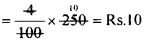Bill amount = 250+10 = Rs. 260.

(b) Marked price is Rs. 2580 and sale tax 10%
Tax =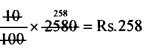Bill amount = 2580 + 258 = Rs. 2838.

(c) Marked price Rs. 1200 and tax 3%
Tax =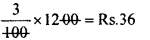Bill amount = 1200 + 36 = Rs. 1236.

(d) Marked price Rs. 200 and sales tax 6%
Tax =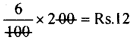Bill amount = 200 + 12 = Rs. 212.

Question 2.
A person buys electronic goods worth Rs. 10,000 for which the sales tax is 4% and other material worth Rs. 15,000 for which the sales tax is 6%. He manufactures a gadget using all these and sells it at 15% profit, what is his selling price?
Price of the electronic goods is Rs. 10,000 and tax is 4%
∴ Tax =4100 × 10,000 = Rs.400
Total cost = 10,000 + 400 = Rs. 10,400
Price of the other material is 15,000 and tax is 6%.
∴ Tax = 6100 × 15,000 = Rs.900
Total cost = 15,000 + 900 = Rs. 15,900
Total cost price of the electronic gadget prepared by him is (10400 + 15900) = 26300
Profit percent = 15%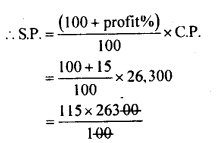selling price = Rs. 30,245

Question 3.
A trader purchases 70 kg of tea at the rate of Rs. 200 kg and another 30kg at the rate of Rs. 250/kg. He pays a sales tax of 4% on the transaction. He mixes both of them and sells the product at the rate of Rs. 240/kg. What is the percentage gain or loss (find approximate value)?
The cost of 70 kg of tea at the rate Rs. 200/ kg is 200 x 70 = Rs. 14000
The cost of 30kg of tea at the rate Rs 250/ kg is 250 x 30 = Rs 75000.
Total cost of 100kg = 14000+7500 = Rs. 21,500
Rate of tax 4%
∴ Tax = 4100 × 21,5000 = Rs.860
Total cost price = 21,500 + 860 = Rs. 22,360
S.P of 100kg tea at Rs. 240/Kg
= 100 x 240 = Rs. 24,000
S.P > C.P
Profit = S.P – C.P
= 24,000 – 22360 = Rs. 1640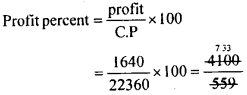Profit percent = 7.33%

All Chapter KSEEB Solutions For Class 8 maths

—————————————————————————–

All Subject KSEEB Solutions For Class 8

*************************************************

I think you got complete solutions for this chapter. If You have any queries regarding this chapter, please comment on the below section our subject teacher will answer you. We tried our best to give complete solutions so you got good marks in your exam.

If these solutions have helped you, you can also share kseebsolutionsfor.com to your friends.

Best of Luck!!